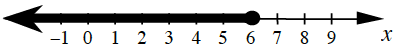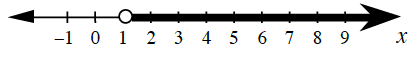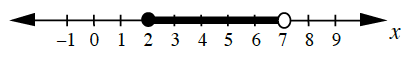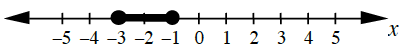### Home > INT1 > Chapter 9 > Lesson 9.3.3 > Problem9-92

9-92.

Write the inequality that represents the $x$-values highlighted on each number line below.

1.The boundary point is $6$.

The arrow is pointing in the direction of numbers less than $6$.

The circle is filled in so the inequality includes $6$.

$x\le6$

1.Follow the steps in part (a).

1.The highlighted $x$-values are greater than or equal to $2$ less than $7$.

$2\le x<7$

1.Follow the steps in part (c).